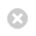Close

Institute of Communications and Connected Systems

Home# Interference Exploitation Precoding for Multi-Level Modulations: Closed-Form Solutions

IEEE Transactions on Communications | Li A, Masouros C, Vucetic B, Li Y, Swindlehurst AL | We study closed-form interference-exploitation precoding for multi-level modulations in the downlink of mu...

1 January 2021

## Abstract

We study closed-form interference-exploitation precoding for multi-level modulations in the downlink of multi-user multiple-input single-output (MU-MISO) systems. We consider two distinct cases: first, when the number of served users is not larger than the number of transmit antennas at the base station (BS), we mathematically derive the optimal precoding structure based on the Karush-Kuhn-Tucker (KKT) conditions. By formulating the dual problem, the precoding problem is transformed into a pre-scaling operation using quadratic programming (QP) optimization. We further consider the case where the number of served users is larger than the number of transmit antennas at the BS. By employing the pseudo inverse, we show that the optimal solution of the pre-scaling vector is equivalent to a linear combination of the right singular vectors corresponding to zero singular values, and derive the equivalent QP formulation. We also present the condition under which multiplexing more streams than the number of transmit antennas is achievable. For both considered scenarios, we propose a modified iterative algorithm to obtain the optimal precoding matrix, as well as a sub-optimal closed-form precoder. Numerical results validate our derivations on the optimal precoding structures for multi-level modulations, and demonstrate the superiority of interference-exploitation precoding for both scenarios.

Publication Type: Journal article Article Li A, Masouros C, Vucetic B, Li Y, Swindlehurst AL Institute of Electrical and Electronics Engineers (IEEE) 01/01/2021 291, 308 IEEE Transactions on Communications 69 1 Published 0090-6778 http://dx.doi.org/10.1109/TCOMM.2020.3031616

Explore how UCL research is advancing the future technologies of a connected world: